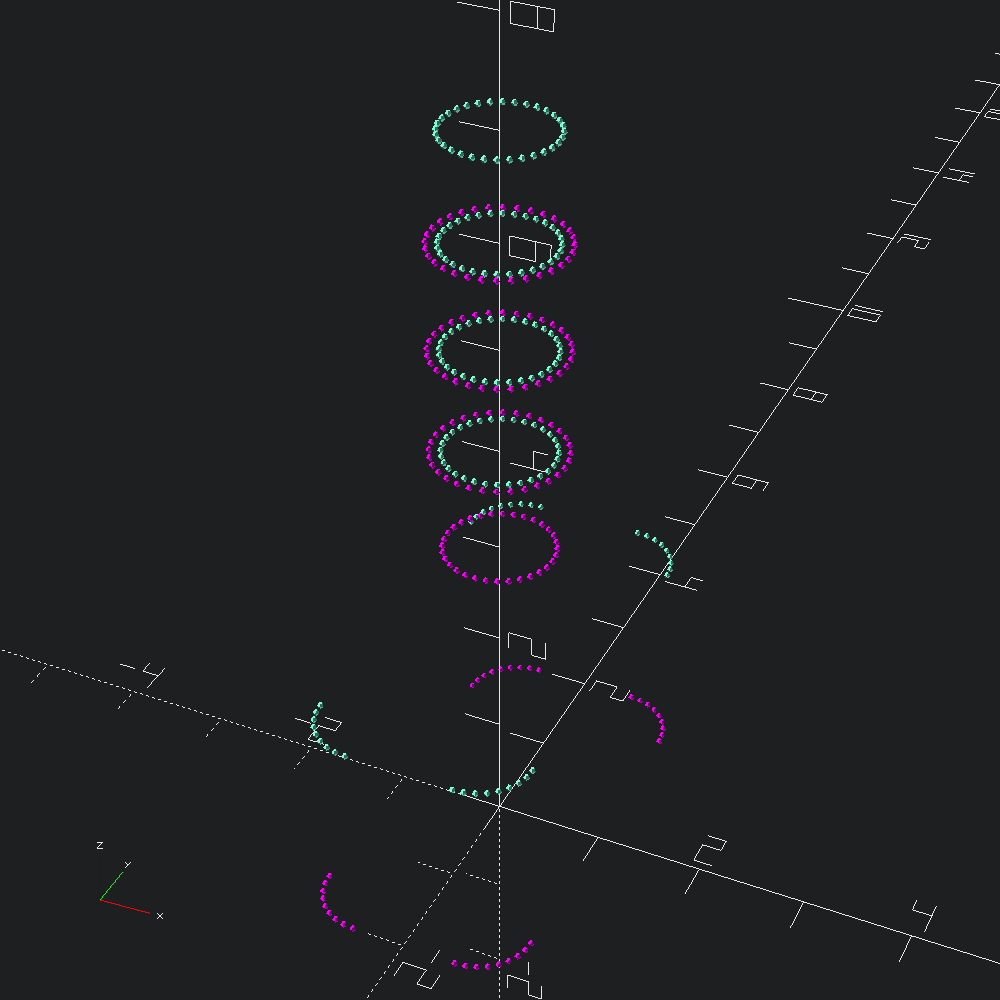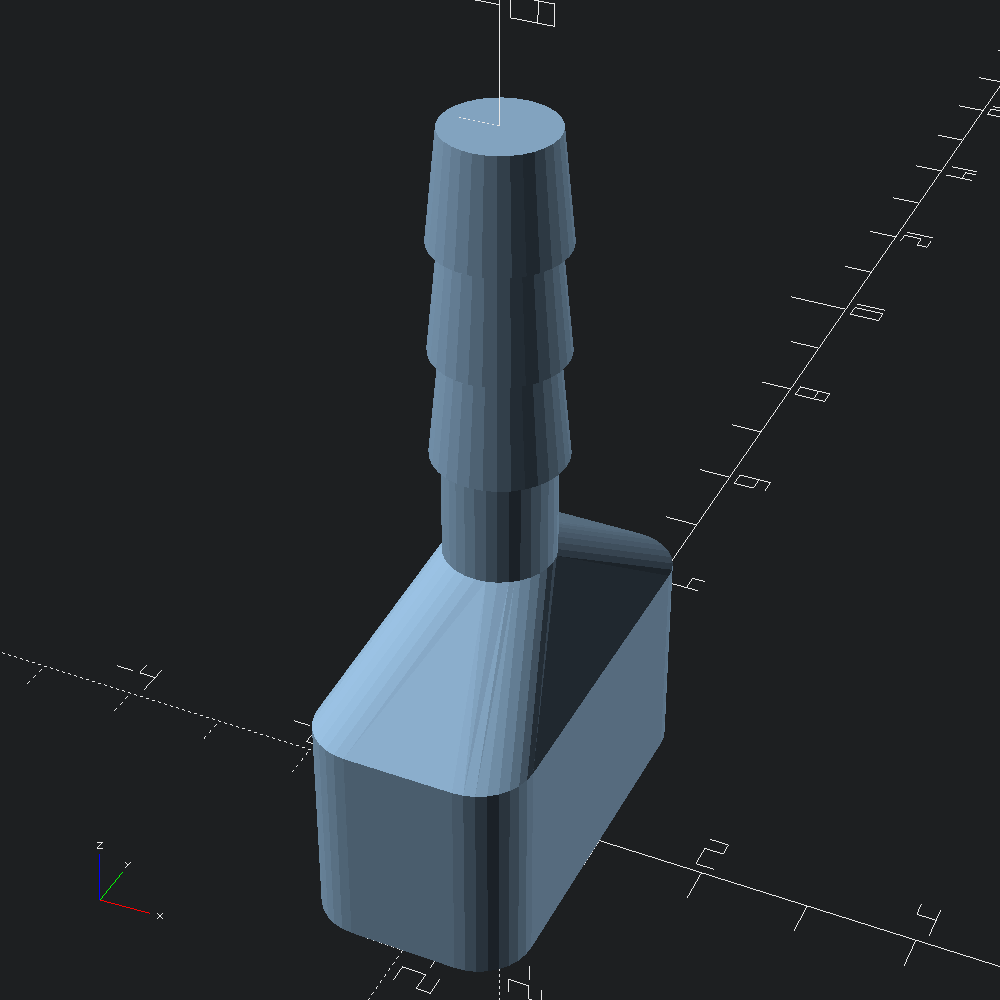# Profile Skinning

``````open OCADml

The `Mesh.skin` function makes it (relatively) simple to generate meshes that cover over series of profiles. A greate simple example demonstrated by the skin modules in both list-comprehension-demos and BOSL2 is the vaccum connector.

``````let profiles =
let fn = 32 in
let base =
let sq = Path3.square ~center:true (v2 2. 4.) in
Path3.(roundover ~fn (Round.flat ~corner:(Round.circ (`Radius 0.5)) sq))
and c r h = Path3.ztrans h @@ Path3.circle ~fn r in
let cones = List.map (fun h -> [ c 0.6 h; c 0.5 (h +. 1.) ]) [ 4.; 5.; 6. ] in
List.flatten ([ base; Path3.ztrans 2. base; c 0.5 3.; c 0.5 4. ] :: cones)``````

A quick look at the points of our profiles we are about to mesh over with alternating colours may help a bit to conceptualize what we are about to give `Mesh.skin` to work with.

``````let () =
let show i =
let c = if i mod 2 = 0 then Color.Magenta else Color.Aquamarine in
Debug.show_path3 (fun _ -> Scad.(color c @@ sphere 0.03))
in``````let () =